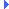FD.io VPP  v18.01-8-g0eacf49 Vector Packet ProcessingInclude dependency graph for load_balance_map.h:This graph shows which files directly or indirectly include this file:

Go to the source code of this file.

## Functions

the state of a path has changed (it has no doubt gone down). More...

static u16 load_balance_map_translate (index_t lbmi, u16 bucket)

## Variables

The encapsulation breakages are for fast DP access. More...

## Function Documentation

 u8* format_load_balance_map ( u8 * s, va_list * ap )

Definition at line 91 of file load_balance_map.c.Here is the call graph for this function:Here is the caller graph for this function:

 static const dpo_id_t* load_balance_get_fwd_bucket ( const load_balance_t * lb, u16 bucket )
inlinestatic

Definition at line 88 of file load_balance_map.h.Here is the call graph for this function:Here is the caller graph for this function:

Definition at line 427 of file load_balance_map.c.Here is the call graph for this function:Here is the caller graph for this function:

inlinestatic

Definition at line 71 of file load_balance_map.h.Here is the caller graph for this function:

 void load_balance_map_lock ( index_t lmbi )

Definition at line 454 of file load_balance_map.c.Here is the call graph for this function:

Definition at line 522 of file load_balance_map.c.Here is the call graph for this function:Here is the caller graph for this function:

 void load_balance_map_path_state_change ( fib_node_index_t path_index )

the state of a path has changed (it has no doubt gone down).

This is the trigger to perform a PIC edge cutover and update the maps to exclude this path.

Definition at line 503 of file load_balance_map.c.Here is the call graph for this function:Here is the caller graph for this function:

Definition at line 537 of file load_balance_map.c.Here is the call graph for this function:Here is the caller graph for this function:

 static u16 load_balance_map_translate ( index_t lbmi, u16 bucket )
inlinestatic

Definition at line 77 of file load_balance_map.h.Here is the call graph for this function:Here is the caller graph for this function:

 void load_balance_map_unlock ( index_t lbmi )

Definition at line 464 of file load_balance_map.c.Here is the call graph for this function:Here is the caller graph for this function: